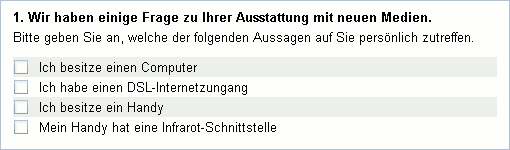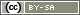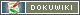This translation is older than the original page and might be outdated. See what has changed.

It may be necessary to link multiple conditions for a filter (Filters and Conditional Questions). Some examples of these links:

• Did the respondent select the option “fairly unimportant” (2) or “fairly important” (3), but not “completely unimportant (1) or “particularly important” (4)?
• Did the respondent state that he owned a car and it has a gas engine?
• Does the respondent neither watch the news on TV nor read it in the paper?
• Does the participant usually buy – according to a multiple-choice – chocolate and dairy products?

These “logical operators” (also known as “Boolean operators”, see PHP: Logical Operators) aid the linking of conditions. Three operators are required particularly frequently:

• The AND operator (`and` or `&&`) links two conditions and checks if both conditions are true.
• The OR operator (`or` or `||`) also links two conditions and checks if at least one of the conditions is true.
• The NOT operator (`not` or `!`) is preceded with a condition. It checks if this condition is not true.

Note: You can only link conditions with the operators; not the values directly:

```(value('AB01') == 1 or 2)  // does not return an error as such, but also does not return the desired result
(value('AB01') == 1) or (value('AB01') == 2)  // correct linking of two conditions```

Suppose the user should be asked whether he has downloaded images, ringtones etc from his cell phone onto the computer before. However, this should only be asked if he owns a computer as well as a cell phone. The user is asked what he owns in a multiple-choice question “TF02”. Options chosen in the multiple choice are given the code 2, those that are not chosen are given the code 1.The individual conditions would be checked as follows:

• `value('TF02_01') == 2` (owns a computer)
• `value('TF02_03') == 2` (owns a cell phone)

An `and` is put in between both conditions. The conditions can be put in brackets so that it stays clear:

`(value('TF02_01') == 2) and (value('TF02_03') == 2)`

In this example, the inner brackets would not be essential because the `==` before the `and` is executed (similar to performing an order of operation calculation). However, using brackets plays it safe when there are complicated constructions.

In IF filters, the whole condition is put in round brackets again (because the condition is always put in brackets when using the IF function). A filter with the linked condition therefore looks as follows:

```if ((value('TF02_01') == 2) and (value('TF02_03') == 2)) {
question('IN20');  // data transfer cell phone-computer
}```

If the bracket structure is no longer clear, multiples lines can also easily be used. The following PHP code has the exact same function as the example above:

```if (
(value('TF02_01') == 2) and
(value('TF02_03') == 2)
) {
question('IN20');  // data transfer cell phone-computer
}```

If more than two conditions should be linked then indentations offer clarity – and thus prevent mistakes.

## The One OR the Other Condition is True

The following example shows a check as to whether at least one of the first three items from the question displayed above was chosen (owns a computer, DSL connection or cell phone). The “or” operator is used here in order to do so. In the event that none of the sub-conditions are true, the questionnaire will jump to the end.

```if (
(value('TF02_01') == 2) or
(value('TF02_02') == 2) or
(value('TF02_03') == 2)
) {
// display a text on the screen
html('<p>Select at least one of the three options!</p>');
} else {
// none selected: end survey
goToPage('end');
}```

Tip: Three or four items in a question can be called up in the same way. However, there is a more elegant solution once there are more items. This is introduced in the chapter Check If at Least One Value was Selected.

## Check If a Selection has the One OR Another Value

It must often be checked if a certain set of values was selected in a completely normal selection or for a scale item. For example, if the value 1, 2 or 3 was selected.

If the values are directly linked, the greater than (>) and less than (<) comparison operators can be used.

```if ((value('AB01') > 0) and (value('AB01') < 4)) {
question('BB02');
}```

If the values are not linked, then each condition can be called up individually:

```if (
(value('AB01') == 1) or
(value('AB01') == 2) or
(value('AB01') == 3)
) {
question('BB02');
}```

In order to make the PHP code more clear, the value can be saved in a PHP variable first of all:

```\$a1 = value('AB01');
if ((\$a1 == 1) or (\$a1 == 2) or (\$a1 == 3)) {
question('BB02');
}```

Arrays offer an alternative for a larger number of values, in conjunction with PHP's own function `in_array()`.

```if (in_array(value('AB01'), array(1,2,3))) {
question('BB02');
}```

## Check If a Condition is NOT True

The NOT operator (`not` or `!`) checks to see if a condition is not true. This means the respondent can be sent to the end of the questionnaire if he does not own a computer:

```if (not (value('TF02_01') == 2)) {
goToPage('end');
}```

However, the not equal comparison operator (`!=`) is used more for this filter:

```if (value('TF02_01') != 2) {
goToPage('end');
}```

If it is known that `TF02_01` can only accept the values 1 and 2 anyway, then the filter can be set even more simply:

```if (value('TF02_01') == 1) {
goToPage('end');
}```

However, there are definitely cases where the NOT operator is helpful. Taking the OR example from above into account: if the respondent neither owns a PC, nor a DSL connection or cell phone, he should be sent directly to the end of the questionnaire:

```if (!(
(value('AB01') == 1) or
(value('AB01') == 2) or
(value('AB01') == 3)
)) {
goToPage('end');
}```

The only difference between this and the OR example above is the exclamation point, which is equivalent to `not`, and the additional brackets at the beginning and the end.

In addition, there is a mathematical distributive law when calculating with the NOT operator. The following filter has the same effect as the previous one:

```if (
(value('AB01') != 1) and
(value('AB01') != 2) and
(value('AB01') != 3)
) {
goToPage('end');
}```

Of course, just the `else` part of the IF construction can also be used:

```if (
(value('AB01') == 1) or
(value('AB01') == 2) or
(value('AB01') == 3)
) {
// do nothing
} else {
goToPage('end');
}```